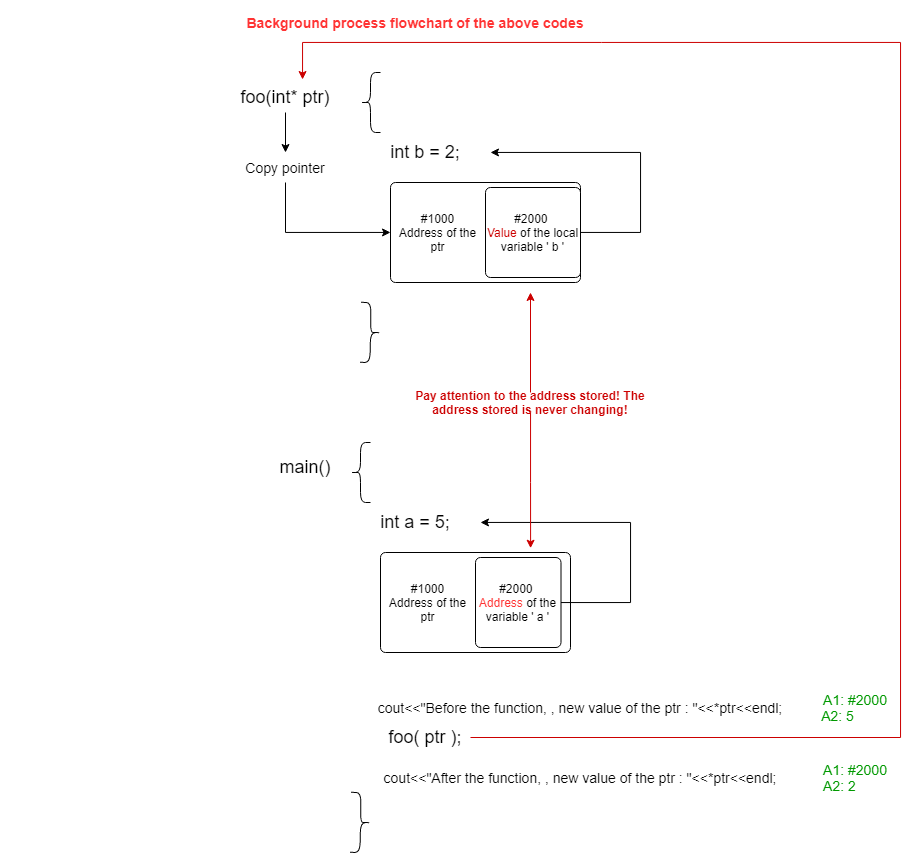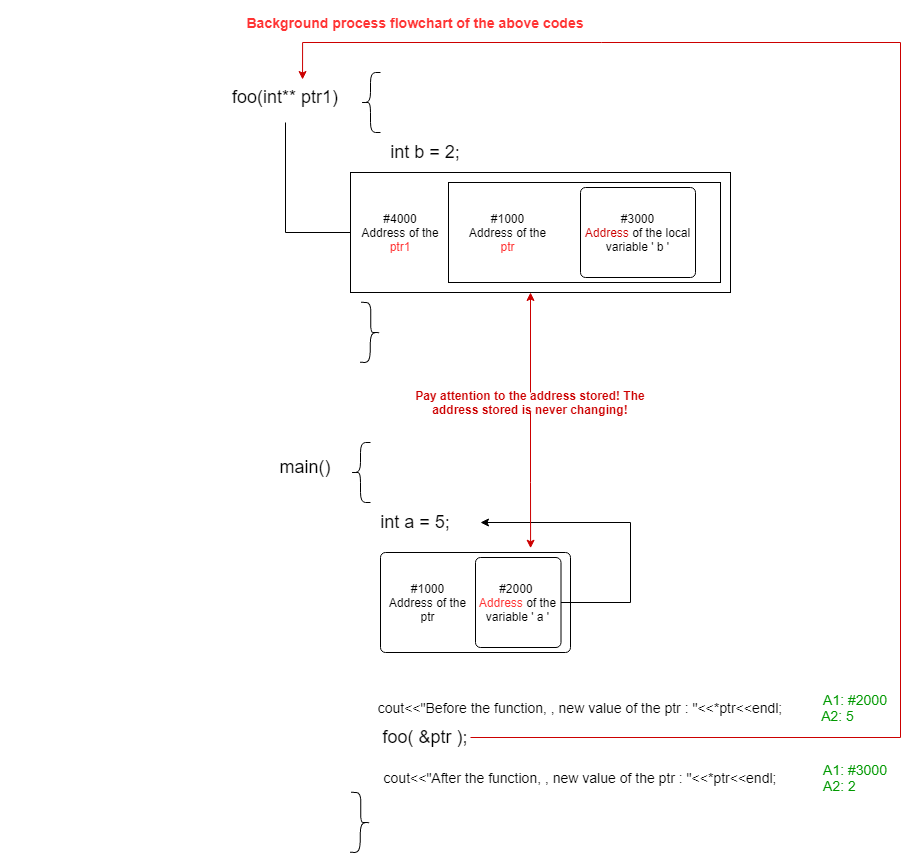GeeksforGeeks App
Open AppBrowser
Continue

# Difference between passing pointer to pointer and address of pointer to any function

In this article, the differences between passing “pointer to pointer” and “address of pointer” to a function. In C or C++ Programming Language, it is known that pointers hold the address of the variables or any memory location. If pointers are pointed to the memory location, it can be used to change the value of the variable.

As for functions, any pointer can be passed by itself, or by the address of the pointer. But If the memory location of the pointer is to be changed permanently out of the function, it must be captured with a reference or double-pointer within the function i.e., with the assumption any memory is created inside the local function.

Afterward, it is pointed to the pointer captured by the function without any reference or any double-pointer. In this situation, there is no change inside the main function because it was not captured by reference or a double-pointer inside the function definition.

Program 1:

## C++

 `// C++ program to illustrate the``// concepts pointers` `#include ``#include ``using` `namespace` `std;` `// Function to assign the value of``// pointer ptr to another location``void` `foo(``int``* ptr)``{``    ``int` `b = 2;` `    ``// ptr= (int*)malloc(sizeof(int));``    ``// It can be executed instead of``    ``// the ptr=&b but nothing change``    ``ptr = &b;``}` `// Driver Code``int` `main()``{``    ``int` `a = 5;``    ``int``* ptr = &a;` `    ``cout << ``"Before the function ptr: "``         ``<< *ptr << endl;``    ``foo(ptr);` `    ``cout << ``"After the function ptr: "``         ``<< *ptr << endl;` `    ``return` `0;``}`

Output:

```Before the function ptr: 5
After the function ptr: 5```

Explanation: The reason why there is no change in the output is that it is created a copy pointer for the function. So, the change in the address of the pointer does not reflect out of the function. References or double-pointer can be used for this.

Program 2:

## C++

 `// C++ program to illustrate the``// concepts of pointer` `#include ``#include ``using` `namespace` `std;` `// Function to change the pointer ptr``void` `foo(``int``* ptr)``{``    ``int` `b = 2;` `    ``// The change will only be for``    ``// the new value of the ptr``    ``*ptr = b;``}` `// Driver Code``int` `main()``{` `    ``int` `a = 5;``    ``int``* ptr = &a;` `    ``cout << ``"Before the function, "``         ``<< ``"new value of the ptr: "``         ``<< *ptr << endl;` `    ``// Function Call``    ``foo(ptr);` `    ``cout << ``"After the function, "``         ``<< ``"new value of the ptr: "``         ``<< *ptr << endl;` `    ``return` `0;``}`

Output:

```Before the function, new value of the ptr: 5
After the function, new value of the ptr: 2```

Explanation:If it is wanted to replace the memory stored in ptr when the foo() function is complete, ptr must be captured with the reference or double-pointer in the foo() function.

Program 3:

## C++

 `// C++ program to illustrate the``// concepts of pointer``#include ``#include ``using` `namespace` `std;``int` `b = 2;` `// Function to change the value of``// the pointers ptr1``void` `foo(``int``** ptr1) { *ptr1 = &b; }` `// Driver Code``int` `main()``{``    ``int` `a = 5;``    ``int``* ptr = &a;` `    ``cout << ``"Before the function, "``         ``<< ``"new value of the ptr: "` `<< *ptr << endl;` `    ``// Function Call``    ``foo(&ptr);` `    ``cout << ``"After the function, "``         ``<< ``"new value of the ptr: "` `<< *ptr << endl;` `    ``return` `0;``}`

Output

```Before the function, new value of the ptr: 5
After the function, new value of the ptr: 2
```

Explanation:My Personal Notes arrow_drop_up